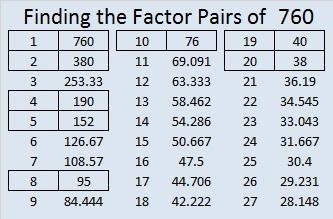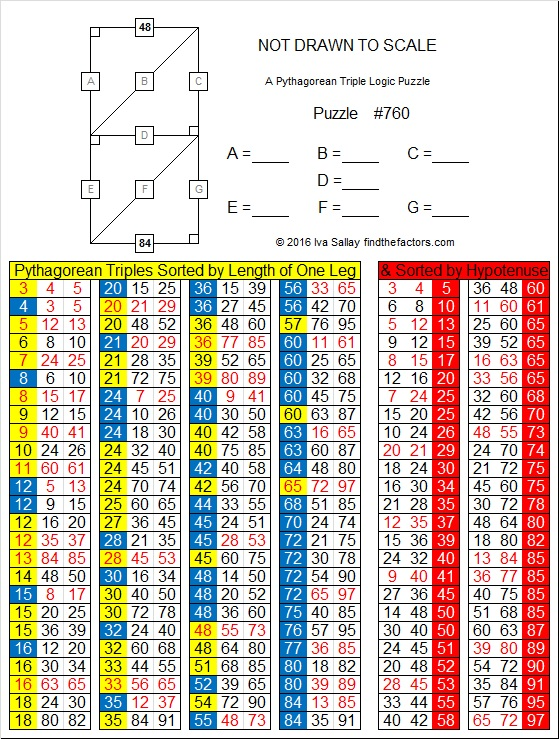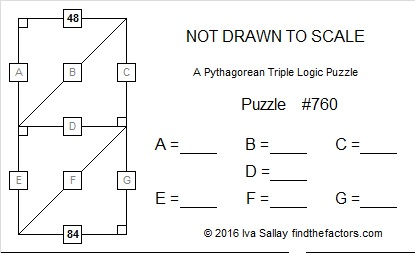# 760 Not a Misprint: One side is 84, the other 48. What are the other sides?

• 760 is a composite number.
• Prime factorization: 760 = 2 x 2 x 2 x 5 x 19, which can be written 760 = (2^3) x 5 x 19
• The exponents in the prime factorization are 3, 1, and 1. Adding one to each and multiplying we get (3 + 1)(1 + 1)(1 + 1) = 4 x 2 x 2 = 16. Therefore 760 has exactly 16 factors.
• Factors of 760: 1, 2, 4, 5, 8, 10, 19, 20, 38, 40, 76, 95, 152, 190, 380, 760
• Factor pairs: 760 = 1 x 760, 2 x 380, 4 x 190, 5 x 152, 8 x 95, 10 x 76, 19 x 40, or 20 x 38
• Taking the factor pair with the largest square number factor, we get √760 = (√4)(√190) = 2√190 ≈ 27.5680975.There is no misprint in this puzzle. One side really is 84 while the other side really is 48. Can you find the other sides?Print the puzzles or type the solution on this excel file: 10 Factors 2016-02-04

—————————————

Here’s more about the number 760:

760 is the sum of consecutive numbers two different ways. (Two of its factor pairs show up in those ways.):

• 150 + 151 + 152 + 153 + 154 = 760; that’s 5 consecutive numbers.
• 31 + 32 + 33 + 34 + 35 + 36 + 37 + 38 + 39 + 40 + 41 + 42 + 43 + 44 + 45 + 46 + 47 + 48 + 49 = 760; that’s 19 consecutive numbers.

760 is the hypotenuse of a Pythagorean triple so that 456² + 608² = 760²

760 is the sum of three squares: 20² + 18² + 6² = 760.

760 is palindrome 1A1 in Base 23 because 1(23²) + 10(23) + 1(1) = 760.

Wikipedia informs us that 760 is the 23rd centered triangular number because (3⋅22² + 3⋅22 + 2)/2 = 760.

—————————————

Here’s the same puzzle with out the lists of triples: